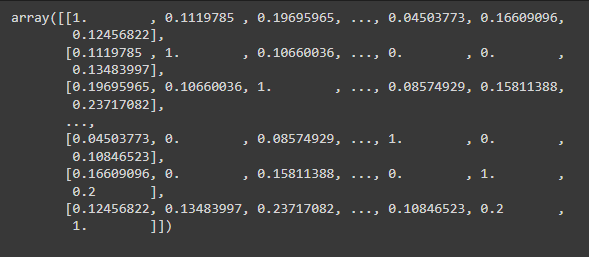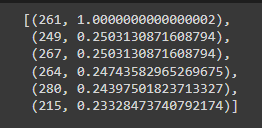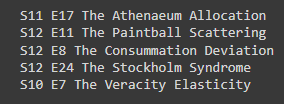Mani Garg — Published On March 1, 2022

This article was published as a part of the Data Science Blogathon.

(The dataset is freely available to the public for use. Also, the file is in .xlsx format, you can convert it to csv file by simply saving it in that format).

Let’s Start!!

## Data Preparation and Cleaning

You will rarely come across a dataset that you can use as it is i.e. the dataset will be not ready to use, without you transforming.

Let’s first start by importing the required libraries and uploading our dataset. You can start a new Jupyter notebook or use a Google collab notebook for this purpose.

Let’s start by installing and importing Pandas and Numpy libraries of python.

```#installing pandas and numpy
!pip install pandas — upgrade — quiet
!pip install numpy — upgrade — quiet
#importing pandas and numpy
import pandas as pd
import numpy as np```

It’s good to import pandas as pd and numpy as np because it is easier to use them.

Now let’s read our dataset by using the name of the csv file that we saved. You will need to upload the dataset file first in the files section of the jupyter notebook or google collab.

```#reading the dataset

The reason that I named the file as df is that Pandas stores the file as dataframe, which is a data type just like a string, int, etc.

Now let’s see how our data looks by viewing the first few rows of our data using head().

`df.head()`

Now let’s see how many rows and columns do we have in our dataset by using shape().

`df.shape()`

Now let’s see the basic information about the data, like which type is each column, etc.

`df.info()`

If you are thinking about whether you need to memorize all of these various functions like head, shape, etc. then the answer is No, you will know what to use with practice, but for the time being, just search on the internet what you want to achieve with your data and surely you will find an answer.

Whenever you clean your data there are some things that you need to generally do like checking for null values and replacing them with the appropriate value and finding duplicate values and eliminating them.

`df.duplicated().sum()`
`df.isna().sum()`

As you might have seen that in our data Season and Episodes are just individual numbers, I am going to add “S” and “E” in Season and Episode Number, just to make it look a bit more presentable.

It is as simple as concatenating two strings using the ‘+’ operator.

```df[‘Season’] =’S’ + df[‘Season’].astype(str)
df[‘Episode Number’] =’E’ + df[‘Episode Number’].astype(str)```

Let’s see how it looks

`df.head()`

Perfect!

And overall our dataset looks pretty clean, so let’s do some EDA.

## Exploratory Analysis and Visualization

We will also generate some charts and graphs along the way, so for that let’s import matplotlib and seaborn library.

```import seaborn as sns
import matplotlib
import matplotlib.pyplot as plt
%matplotlib inline
sns.set_style(‘darkgrid’)
matplotlib.rcParams[‘font.size’] = 14
matplotlib.rcParams[‘figure.figsize’] = (9, 5)
matplotlib.rcParams[‘figure.facecolor’] = ‘#00000000’```

Don’t worry about each line of the above code it just set some basic things like chart size, font size, etc. You can only import the libraries using the first three lines of the code.

Let’s see how many episodes are there per season, for that we will use value_counts(). It returns the total values according to a specified column in this case Season. Sorting is set to false because we want the result season-wise and not in descending order which is what is given by default.

```episode_per_season = df.Season.value_counts(sort=False)
episode_per_season```

Now let’s create a bar chart for this using seaborn.

`sns.barplot(x = episode_per_season.index, y = episode_per_season);`

Again you can simply search the internet for its syntax until you get the hang of it. Let’s see the names of the top 10 and bottom 10 episodes according to ratings.

```top_10_rated_episodes = df.sort_values(‘Rating’, ascending = False).head(10)
top_10_rated_episodes.Title```

Let’s do one thing, let’s combine season np. Episode no. and title into one column, so that it’s easier to navigate while watching.

```df[‘SE_Title’] = df[‘Season’]+” “+ df[‘Episode Number’]+” “+df[‘Title’]
df[‘SE_Title’]```
```least_10_rated_episodes = df.sort_values(‘Rating’, ascending = True).head(10)
least_10_rated_episodes.SE_Title```

It looks much better now.

Let’s see the average rating of each season.

`Rating_by_season = df.groupby(‘Season’, as_index=False, sort=False).agg({‘Rating’: [‘mean’]})`

Since it was is created as a result of our calculation, this dataframe does not have column names. But we can give columns names using.

```Rating_by_season .columns = [‘season’, ‘Rating’]
Rating_by_season```

And plot it in a line chart.

`sns.lineplot(x=’Season’, y=’Rating’, data=Rating_by_season);`

Rating of the whole show

```Averge_rating_of_the_show = df.agg({‘Rating’: [‘mean’]})
Averge_rating_of_the_show```

## Recommendation System

There are two types of recommendation system models content-based and collaborative based you can more about them here. But in this model, we will use content-based, which means If you watched the movie “A” and according to the plot movie “B” is similar to “A”, then “B” will be recommended to you to watch.

In our dataset, we are given a plot of each episode, so we are going to use it to check similarity between episodes,

We will import the required scikit libraries for this

```from sklearn.feature_extraction.text import CountVectorizer
#stop_words attribute will skip all the helper words that occur english language eg: in, the , they etc.
cv_fvector = CountVectorizer(stop_words=’english’)
vector = cv_fvector.fit_transform(df[‘Plot’]).toarray()```

Here we have imported count vectorizer. Since machines can’t understand the text as we do, this library converts the text into numerical data (in this case vectors). How it does that is, it counts the frequency of each distinct word that occurs in a sentence. For instance:

Sample = [“This is cat”, “This is dog”]

And then we used fit_transform to autoscale the data.

```from sklearn.metrics.pairwise import cosine_similarity
similarity_array = cosine_similarity(vector)
similarity_array```screenshot of the output

It will give us an array calculating the cosine distance of each vector with the other vector.Let’s test our model

```episode = 'S12 E5 The Planetarium Collision'
//this gives the index no. of the episode in our data set```
`eps_index = df[df['SE_Title'] == episode].index`

Now we will find the top five episodes’ index and the similarity with our episode

```similar_eps = sorted(list(enumerate(similarity[eps_index])),reverse=True,key = lambda x: x)
similar_eps[0:6]```

Lastly, we use a very simple for loop to fetch the names of our results

```for i in similar_eps[1:6]:
print(df.iloc[i].SE_Title)```screenshot of the output

This was the final result that I got.

## Conclusion

We performed some EDA and made a pretty decent recommendation system. You can still do a lot of things with this dataset. I didn’t use the “votes” column, you can use it to generate some useful insights. You can make the “Plot” column, a bit more relevant for the recommendation system, by adding ratings, season number, etc. to it. Just think about what could be possible and there will be a way to achieve the desired result.  There are so many awesome datasets available, use them and you will find some very interesting insights. You can download any freely available dataset on movies, books, etc, and make your very own recommendation system. Don’t Stop now.